Software Engineering
Volume 3, Issue 3, September 2015, Pages: 12-17

Modified Method for One-Dimensional Cutting Stock Problem

Niluka Rodrigo, WB Daundasekera, AAI Perera

Department of Mathematics, Faculty of Science, University of Peradeniya, Peradeniya, Sri Lanka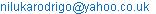(N. Rodrigo)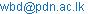(WB Daundasekera)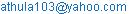(AAI Perera)

Niluka Rodrigo, WB Daundasekera, AAI Perera. Modified Method for One-Dimensional Cutting Stock Problem.Software Engineering.Vol.3, No. 3, 2015, pp. 12-17. doi: 10.11648/j.se.20150303.11

Abstract: Selection of feasible cutting patterns in order to minimize the rawmaterial wastage which is known as cutting stock problem has become a key factor of the success in today’s competitive manufacturing industries. In this paper, solving a one-dimensional cutting stock problem is discussed. Our study is restricted to rawmaterial (main sheet) in a rectangular shape (different sizes), and cutting items are also considered as rectangular shape with known dimensions (assume that lengths of the main sheets and cutting items are equal). Pattern generation technique is used to nest the pieces of cutting items within the main sheet by minimizing rawmaterial wastage. A computer program using Matlab software package is developed to generate feasible patterns using the above algorithm for 1D cutting stock problem. Location of each feasible cutting pattern inside the main sheet is given in Cartesian Coordinate Plane. The Branch and Bound approach in solving integer programming problems is used to solve the problem.

Keywords: Cutting Stock Problem, Branch and Bound Algorithm, Pattern Generation, Matlab Software Package

Contents

1. Introduction

Competence of production system becomes a key factor of the success in today’s competitive manufacturing environment and industries. Productivity can be enhanced by minimizing waste, lead time and hence reducing the cost of manufacture. For that reason, Operations Research plays a major role in minimizing manufacture waste. Many people including scientists have contributed and engaged in their research and other activities to overcome above challenges. A cutting stock problem basically describes in two ways, One-dimensional (1D) and Two-dimensional (2D) cutting stock problems. It consists of cutting large pieces, which are available in stock with different shapes to produce smaller pieces which are known as items, in order to meet a given demand. This often results in smaller pieces which cannot be used for the production and considered as waste. So, cutting items should be designed to minimize waste of rawmaterials. Many researchers have worked on the cutting stock problem and developed different algorithms to solve the problem. Among them, Gilmore et al  conducted some of the earliest research in this area and one-dimensional cutting stock problem is solved using Linear Programming Technique. In this study, unlimited numbers of raw materials with different lengths are assumed available in stock, and a mathematical model has been developed to minimize the total cutting cost of the stock length of the feasible cutting patterns and Column Generation Algorithm has been developed to generate feasible cutting patterns. Then, Gilmore has claimed that feasible cutting patterns are increased with the required cutting items and Linear Programming Technique is not applicable to solved mathematical model with too many variables. Gilmore  has made an approach for one-dimensional cutting stock problem as an extended of earliest paper (Gilmore et al (1961)) and cutting stock problem has been described as a NP-hard problem. A new and rapid algorithm for the knapsack problem and changes in the mathematical formulation1 has been evolved and Gilmore has explained the procedure of the Knapsack Method using a test problem. Saad M.A Suliman  has modified Branch and Bound Algorithm to find feasible patterns for 1D cutting stock problem. In the case study, Saad has selected four different types of steel coils to cut from the standard steel coil with the 130 cm length and width of the main coil and widths of the required coils are equal. Branch and Bound Algorithm has been explained using the example. Also, Sirirat and Peerayuth  has proposed a mathematical model with column generation technique by a Branch and Bound procedure and Heuristic based on the First Fit decreasing method for one-dimensional cutting stock problem. Sirirat has made assumptions that different lengths of items to be cut from a stock, Each item has associated a certain length, and each cutting pattern for a stock are not limited in the number of knives, but the sum of the length of items do not exceed a length of stock. These problems arise in industries such as garment, paper, glass etc. Beasley  has discussed the unconstrained two-dimensional cutting stock problem with guillotine cuts (cuts that start from one edge and parallel to the other two edges) and staged cuts (the cuts at the first stage are restricted to be guillotine cuts parallel to one axis, then the cuts at the second stage are restricted to be guillotine cuts parallel to the other axis and the cuts at the third stage are restricted to be guillotine cuts parallel to the original axis etc.).

There are different arrangements to cut required pieces from the existing raw material to maximize the used area and each arrangement is defined as a cutting pattern. Rodrigo et al  has presented modified Branch and Bound Algorithm and a computer program using Matlab software package to generate feasible cutting patterns. As a case study, 1200 cm long steel bars are considered to be cut into eight types (different lengths) of pieces according to the customer requirements.  Rodrigo et al [7,8] has presented modified Branch and Bound Algorithm for two-dimensional cutting stock problem with fixed size rawmaterial and a computer program using Matlab software package to generate feasible cutting patterns.  Coromoto et al (2007) has used a Parallel Algorithm and Sequential Algorithm to solve the mathematical model which maximizes the total profit incurred by cutting n number of rectangular pieces from a large rectangular main sheet. Coromoto  observed that all cutting patterns can be obtained by means of horizontal and vertical builds of meta-rectangles and has used Viswanathan and Bagchi Algorithm to produce best horizontal and vertical builds.

In this study, modified Branch and Bound Algorithm is further modified to solve the one-dimensional (1D) cutting stock problem with different sizes of rawmaterials. Pattern generation technique is used to reduce the wastage and a computer program using Matlab software package is developed to generate feasible cutting patterns and to define the location of each item in each pattern within the Cartesian coordinate plane for one-dimensional rectangular shape cutting stock problem as an extended of the earliest paper (Rodrigo et al (2011)).

2. Materials and Methods

Any firm’s main goal is to maximize the annual contribution periphery accruing from its manufacture and sales. By reducing wastages and maximizing sales, efficiency can be improved. Wastage can arises in many ways and at any step of production line cutting stock problem can be described under the rawmaterial wastage.

According to the selection, a mathematical model to minimize the rawmaterial wastage is formulated as follows:

m = Number of pieces,

n = Number of patterns,

r = Number of rawmaterials with different sizes,

a i j = The number of occurrences of thepiece in the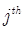pattern of kth rawmaterial,

x jk = The number of main sheets being cut according to thepattern of kth rawmaterial,

cjk = Cutting loss for eachpattern of kth rawmaterial,

di = Demand of thepiece.

2.1. Mathematical Model (Gilmore and Gomory)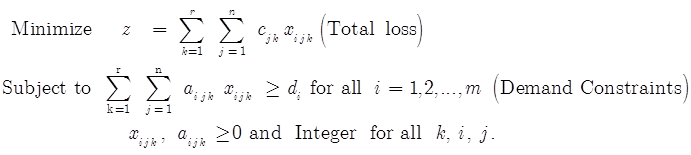The number of occurrences of thepiece in thepattern of kth rawmaterial should be found to find the optimum solution (minimum-waste arrangement) for the above mathematical model. Therefore, Branch and Bound Algorithm (Saad M.A Suliman, 2001) is used to generate feasible cutting patterns.

Here,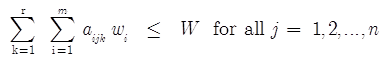,

where wi is the width of theitem and Wk is the width of the kthmain sheet.

A computer program using Matlab software package is developed to generate feasible patterns using the above algorithm for 1D cutting stock problem. Consequently, generated feasible patterns are used to solve the mathematical model given in the paper to obtain the number of main sheets needed to satisfy the requirements of each piece and minimum cutting waste.

2.2. Modified Branch and Bound Algorithm

Step 1: Arrange required widths (or lengths) of rawmaterials, Wk, k = 1, 2, …, r in decreasing order, ie W1 > W2 > … > Wr, where r = number of main sheets.

Step 2: Arrange required lengths, wi, i = 1, 2, …, m in decreasing order, ie w1 > w2 > … > wm ,

where m = number of items.

Step 3: For i = 1, 2,…, m ; k = 1, 2,…, r and j = 1 do Steps 4 to 6.

Step 4: Set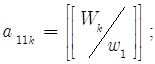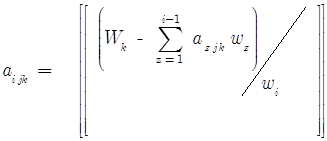(1)

where Wk is the length of the kth main sheet. Here,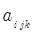is the number of pieces of theitem in thepattern along the width of the kth main sheet and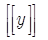is the greatest integer less than or equal to y.

Step 5:where pijk is the number of pieces of the ith item in the jth pattern of the kthmain sheet.

Step 6: Cut loss along the width of the kth main sheet: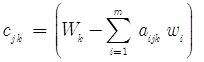Step 7: Set t = m – 1.

While t > 0 , do Step 8.

Step 8: While atjk > 0

set j = j + 1 and do Step 9.

Step 9: Generate a new pattern according to the following conditions: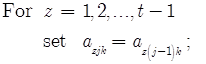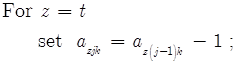For z = t+1, …, m

calculate azjk sing (1).

Go to Step 5.

Step 10: Set t = t – 1.

Step 11: STOP.

2.3. Case Study

Proposed algorithm to solve one-dimensional cutting stock problem with different sizes of rawmaterials is tested and analyzed to determine feasible and optimal cutting patterns. Following example will illustrate how to generate feasible cutting patterns by minimizing total cutting waste:

A paper mill uses paper rolls of width 1000 mm, 500 mm and 800 mm as rawmaterials to cut different sizes paper items according to the given specifications. The company has received an order according to the dimensions given in Table 1:

Table 1. Required item dimensions and demand.

 Item No 1 2 3 4 Required widths 500 210 297 250 (mm) Demand (sheets) 1000 2500 1500 1500

3. Results and Discussion

Modified Branch and Bound Algorithm is applied to the above example to generate feasible cutting patterns as given below:

Step 1: For k = 1, 2, 3 widths of rawmaterials Wk = 1000 mm, 800 mm and 500 mm.

Step 2: For i = 1, 2, 3, 4 widths of items wi = 500 mm, 297 mm, 250 mm and 210 mm.

Step 3: For i = 1, 2… 4 and j = 1 and k = 1, do Steps 4 to 6.

Step 4: Set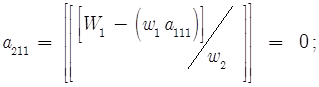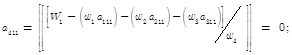Step 5: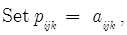Pattern 1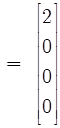Step 6: Total cut loss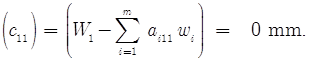Step 7: Set t = 4 – 1.

While t > 0, do Step 8.

Step 8: a311 = 0

Step 8: a211 = 0

Step 8: a111 > 0

Step 9: Generate a new pattern according to the following conditions: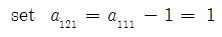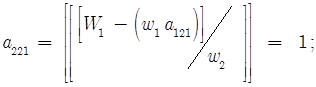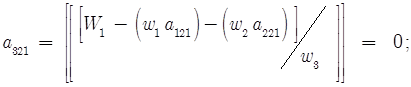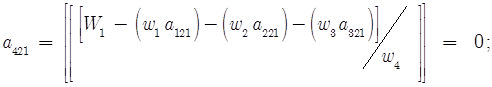Step 5:Pattern 2

Step 6: Total cut loss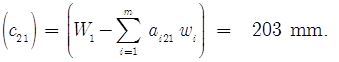The algorithm proceeds in the same manner to generate all the cutting patterns shown in Table 2.

Table 2. Generated cutting patterns.

 Required widths Cutting patterns (from 1000 mm rawmaterial) 1 2 3 4 5 6 7 8 9 10 11 12 13 14 15 16 500 mm 2 1 1 1 1 0 0 0 0 0 0 0 0 0 0 0 297 mm 0 1 0 0 0 3 2 2 1 1 1 0 0 0 0 0 250 mm 0 0 2 1 0 0 1 0 2 1 0 4 3 2 1 0 210 mm 0 0 0 1 2 0 0 1 0 2 3 0 1 2 3 4 Cut Loss (mm) 0 203 0 40 80 109 156 196 203 33 73 0 40 80 120 160 Required widths Cutting patterns (from 800 mm rawmaterial) (from 500 mm) 17 18 19 20 21 22 23 24 25 26 27 28 29 30 31 32 500 mm 1 1 1 0 0 0 0 0 0 0 0 1 0 0 0 0 297 mm 1 0 0 2 1 1 1 0 0 0 0 0 1 0 0 0 250 mm 0 1 0 0 2 1 0 3 2 1 0 0 0 2 1 0 210 mm 0 0 1 0 0 1 2 0 1 2 3 0 0 0 1 2 Cut Loss (mm) 3 50 90 206 3 43 83 50 90 130 170 0 203 0 40 80

# of main sheet = 3

Width of the main sheet = [1000, 800, 500]

Number of pieces = 4

Width of the pieces = [500,297,250,210]

pattern = (1)

Number of pieces of item (1) within (1)st sheet = (2)

Number of pieces of item (2) within (1)th sheet = (0)

Number of pieces of item (3) within (1)th sheet = (0)

Number of pieces of item (4) within (1)th sheet = (0)

Cutloss of pattern (1) = (0)

pattern = (2)

Number of pieces of item (1) within (1)th sheet = (1)

Number of pieces of item (2) within (1)th sheet = (1)

Number of pieces of item (3) within (1)th sheet = (0)

Number of pieces of item (4) within (1)th sheet = (0)

Cutloss of pattern (2) = (203)

pattern = (3)

Number of pieces of item (1) within (1)th sheet = (1)

Number of pieces of item (2) within (1)th sheet = (0)

Number of pieces of item (3) within (1)th sheet = (2)

Number of pieces of item (4) within (1)th sheet = (0)

Cutloss of pattern (3) = (0)

pattern = (4)

Number of pieces of item (1) within (1)th sheet = (1)

Number of pieces of item (2) within (1)th sheet = (0)

Number of pieces of item (3) within (1)th sheet = (1)

Number of pieces of item (4) within (1)th sheet = (1)

Cutloss of pattern (4) = (40)

pattern = (5)

Number of pieces of item (1) within (1)th sheet = (1)

Number of pieces of item (2) within (1)th sheet = (0)

Number of pieces of item (3) within (1)th sheet = (0)

Number of pieces of item (4) within (1)th sheet = (2)

Cutloss of pattern (5) = (80)

pattern = (6)

Number of pieces of item (1) within (1)th sheet = (0)

Number of pieces of item (2) within (1)th sheet = (3)

Number of pieces of item (3) within (1)th sheet = (0)

Number of pieces of item (4) within (1)th sheet = (0)

Cutloss of pattern (6) = (109)

pattern = (7)

Number of pieces of item (1) within (1)th sheet = (0)

Number of pieces of item (2) within (1)th sheet = (2)

Number of pieces of item (3) within (1)th sheet = (1)

Number of pieces of item (4) within (1)th sheet = (0)

Cutloss of pattern (7) = (156)

pattern = (8)

Number of pieces of item (1) within (1)th sheet = (0)

Number of pieces of item (2) within (1)th sheet = (2)

Number of pieces of item (3) within (1)th sheet = (0)

Number of pieces of item (4) within (1)th sheet = (1)

Cutloss of pattern (8) = (196)

pattern = (9)

Number of pieces of item (1) within (1)th sheet = (0)

Number of pieces of item (2) within (1)th sheet = (1)

Number of pieces of item (3) within (1)th sheet = (2)

Number of pieces of item (4) within (1)th sheet = (0)

Cutloss of pattern (9) = (203)

pattern = (10)

Number of pieces of item (1) within (1)th sheet = (0)

Number of pieces of item (2) within (1)th sheet = (1)

Number of pieces of item (3) within (1)th sheet = (1)

Number of pieces of item (4) within (1)th sheet = (2)

Cutloss of pattern (10) = (33)

pattern = (11)

Number of pieces of item (1) within (1)th sheet = (0)

Number of pieces of item (2) within (1)th sheet = (1)

Number of pieces of item (3) within (1)th sheet = (0)

Number of pieces of item (4) within (1)th sheet = (3)

Cutloss of pattern (11) = (73)

pattern = (12)

Number of pieces of item (1) within (1)th sheet = (0)

Number of pieces of item (2) within (1)th sheet = (0)

Number of pieces of item (3) within (1)th sheet = (4)

Number of pieces of item (4) within (1)th sheet = (0)

Cutloss of pattern (12) = (0)

pattern = (13)

Number of pieces of item (1) within (1)th sheet = (0)

Number of pieces of item (2) within (1)th sheet = (0)

Number of pieces of item (3) within (1)th sheet = (3)

Number of pieces of item (4) within (1)th sheet = (1)

Cutloss of pattern (13) = (40)

pattern = (14)

Number of pieces of item (1) within (1)th sheet = (0)

Number of pieces of item (2) within (1)th sheet = (0)

Number of pieces of item (3) within (1)th sheet = (2)

Number of pieces of item (4) within (1)th sheet = (2)

Cutloss of pattern (14) = (80)

pattern = (15)

Number of pieces of item (1) within (1)th sheet = (0)

Number of pieces of item (2) within (1)th sheet = (0)

Number of pieces of item (3) within (1)th sheet = (1)

Number of pieces of item (4) within (1)th sheet = (3)

Cutloss of pattern (15) = (120)

pattern = (16)

Number of pieces of item (1) within (1)th sheet = (0)

Number of pieces of item (2) within (1)th sheet = (0)

Number of pieces of item (3) within (1)th sheet = (0)

Number of pieces of item (4) within (1)th sheet = (4)

Cutloss of pattern (16) = (160)

pattern = (17)

Number of pieces of item (1) within (2)th sheet = (1)

Number of pieces of item (2) within (2)th sheet = (1)

Number of pieces of item (3) within (2)th sheet = (0)

Number of pieces of item (4) within (2)th sheet = (0)

Cutloss of pattern (17) = (3)

pattern = (18)

Number of pieces of item (1) within (2)th sheet = (1)

Number of pieces of item (2) within (2)th sheet = (0)

Number of pieces of item (3) within (2)th sheet = (1)

Number of pieces of item (4) within (2)th sheet = (0)

Cutloss of pattern (18) = (50)

pattern = (19)

Number of pieces of item (1) within (2)th sheet = (1)

Number of pieces of item (2) within (2)th sheet = (0)

Number of pieces of item (3) within (2)th sheet = (0)

Number of pieces of item (4) within (2)th sheet = (1)

Cutloss of pattern (19) = (90)

pattern = (20)

Number of pieces of item (1) within (2)th sheet = (0)

Number of pieces of item (2) within (2)th sheet = (2)

Number of pieces of item (3) within (2)th sheet = (0)

Number of pieces of item (4) within (2)th sheet = (0)

Cutloss of pattern (20) = (206)

pattern = (21)

Number of pieces of item (1) within (2)th sheet = (0)

Number of pieces of item (2) within (2)th sheet = (1)

Number of pieces of item (3) within (2)th sheet = (2)

Number of pieces of item (4) within (2)th sheet = (0)

Cutloss of pattern (21) = (3)

pattern = (22)

Number of pieces of item (1) within (2)th sheet = (0)

Number of pieces of item (2) within (2)th sheet = (1)

Number of pieces of item (3) within (2)th sheet = (1)

Number of pieces of item (4) within (2)th sheet = (1)

Cutloss of pattern (22) = (43)

pattern = (23)

Number of pieces of item (1) within (2)th sheet = (0)

Number of pieces of item (2) within (2)th sheet = (1)

Number of pieces of item (3) within (2)th sheet = (0)

Number of pieces of item (4) within (2)th sheet = (2)

Cutloss of pattern (23) = (83)

pattern = (24)

Number of pieces of item (1) within (2)th sheet = (0)

Number of pieces of item (2) within (2)th sheet = (0)

Number of pieces of item (3) within (2)th sheet = (3)

Number of pieces of item (4) within (2)th sheet = (0)

Cutloss of pattern (24) = (50)

pattern = (25)

Number of pieces of item (1) within (2)th sheet = (0)

Number of pieces of item (2) within (2)th sheet = (0)

Number of pieces of item (3) within (2)th sheet = (2)

Number of pieces of item (4) within (2)th sheet = (1)

Cutloss of pattern (25) = (90)

pattern = (26)

Number of pieces of item (1) within (2)th sheet = (0)

Number of pieces of item (2) within (2)th sheet = (0)

Number of pieces of item (3) within (2)th sheet = (1)

Number of pieces of item (4) within (2)th sheet = (2)

Cutloss of pattern (26) = (130)

pattern = (27)

Number of pieces of item (1) within (2)th sheet = (0)

Number of pieces of item (2) within (2)th sheet = (0)

Number of pieces of item (3) within (2)th sheet = (0)

Number of pieces of item (4) within (2)th sheet = (3)

Cutloss of pattern (27) = (170)

pattern = (28)

Number of pieces of item (1) within (3)th sheet = (1)

Number of pieces of item (2) within (3)th sheet = (0)

Number of pieces of item (3) within (3)th sheet = (0)

Number of pieces of item (4) within (3)th sheet = (0)

Cutloss of pattern (28) = (0)

pattern = (29)

Number of pieces of item (1) within (3)th sheet = (0)

Number of pieces of item (2) within (3)th sheet = (1)

Number of pieces of item (3) within (3)th sheet = (0)

Number of pieces of item (4) within (3)th sheet = (0)

Cutloss of pattern (29) = (203)

pattern = (30)

Number of pieces of item (1) within (3)th sheet = (0)

Number of pieces of item (2) within (3)th sheet = (0)

Number of pieces of item (3) within (3)th sheet = (2)

Number of pieces of item (4) within (3)th sheet = (0)

Cutloss of pattern (30) = (0)

pattern = (31)

Number of pieces of item (1) within (3)th sheet = (0)

Number of pieces of item (2) within (3)th sheet = (0)

Number of pieces of item (3) within (3)th sheet = (1)

Number of pieces of item (4) within (3)th sheet = (1)

Cutloss of pattern (31) = (40)

pattern = (32)

Number of pieces of item (1) within (3)th sheet = (0)

Number of pieces of item (2) within (3)th sheet = (0)

Number of pieces of item (3) within (3)th sheet = (0)

Number of pieces of item (4) within (3)th sheet = (2)

Cutloss of pattern (32) = (80)

optimum

Number of patterns = (32)

There are 32 feasible cutting patterns available to cut rawmaterials with the dimensions 1000 mm, 800 mm and 500 mm into required items. The mathematical model is developed to design generated cutting patterns so that waste (cut loss) will be minimized and the optimum solution to the model is given in Table 3:

Table 3. Optimum Solution.

 Required widths Optimal Patterns Demand 12 17 31 500 mm 0 1 0 1500 297 mm 0 1 0 1500 250 mm 4 0 1 3000 210 mm 0 0 1 500 # of sheets from each pattern 625 1500 500

Cutting location:

Pattern = (12)

Number of pieces of item (1) within (1)th sheet = (0)

Number of pieces of item (2) within (1)th sheet = (0)

Number of pieces of item (3) within (1)th sheet = (4)

Number of pieces of item (4) within (1)th sheet = (0)

Cutloss of pattern (12) = (0)

Pattern = (17)

Number of pieces of item (1) within (2)th sheet = (1)

Number of pieces of item (2) within (2)th sheet = (1)

Number of pieces of item (3) within (2)th sheet = (0)

Number of pieces of item (4) within (2)th sheet = (0)

Cutloss of pattern (17) = (3)

Pattern = (31)

Number of pieces of item (1) within (3)th sheet = (0)

Number of pieces of item (2) within (3)th sheet = (0)

Number of pieces of item (3) within (3)th sheet = (1)

Number of pieces of item (4) within (3)th sheet = (1)

Cutloss of pattern (31) = (40)

4. Conclusion

In this study, a cutting stock problem is formulated as a mathematical model based on the concept of cutting patterns. As given in Table 2, thirty two cutting patterns are generated and only three cutting patterns are selected as given in Table 3 to cut the main sheets according to the requirements. In this case study, the plant assumes that all the extra pieces from each item as wastage. Also, dimensions of cutting items are large and the total cut loss can be decreased if there are smaller cutting items. Identifying the position of each cutting item in each cutting pattern is also crucial to cut the main sheet. But, identification of cutting location is not fulfilled with previous studies. Advantage of this study over the previous studies is cutting locations of each piece of cutting items can be obtained by Cartesian coordinate system.

References

1. P. C. Gilmore and R. E. Gomory, "A Linear Programming Approach to the Cutting Stock Problem", Operations Research, Vol. 9, No. 2 (1961), 849 - 859.
2. Gilmore PC, Gomory RE. A Linear Programming Approach to the Cutting Stock Problem – Part II. Operations Research. 1963;11(6):863–888.
3. Saad M.A Suliman, "Pattern generating procedure for the cutting stock problem",International Journal of Production Economics 74 (2001) 293-301.
4. Sirirat Wongprakornkul and Peerayuth Charnsethikul, "Solving one-Dimensional Cutting Stock Problem with Discrete Demands and Capacitated Planning Objective", Journal of Mathematics and Statistics 6 (2010) 79 – 83.
5. Beasley JE. Algorithms for Unconstrained Two-Dimensional Guillotine Cutting. Journal of Operations Research Science. 1985;36(4):297–306.
6. Rodrigo WNP, Daundasekera WB, Perera AAI, "Pattern Generation for One-Dimensional Cutting Stock Problem", Peradeniya University Research Session (PURSE), 2011.
7. W. N. P Rodrigo, W.B. Daundasekera, A.A.I Perera "Pattern eneration for Two-Dimensional Cutting Stock Problem" International Journal of Mathematics Trends and Technology (IJMTT), Vol. 3 Issue2 (2012), 54-62.
8. W. N. P. Rodrigo, W. B. Daundasekara and A. A. I. Perera "Pattern Generation for Two-Dimensional Cutting Stock Problem with Location",Indian Journal of Computer Science and Engineering (IJCSE), Vol. 3, No 2, April-May 2012, 354-368.
9. Coromoto Leon, Gara Miranda, Casiano Rodriguez, & Carlos Segura, "2D Cutting Stock Problem: A New Parallel Algorithm and Bounds", (www.springerlink.com/index/906t32v338q7u641.pdf), 25th November 2010.
10. Robert W. Haessler and Paul E. Sweeney, "Cutting Stock Problem and Solution Procedures", European Journal of Operational research 54 (1991), 141 – 150.

 Contents 1. 2. 2.1. 2.2. 2.3. 3. 4.
Article ToolsAbstractPDF(216K)